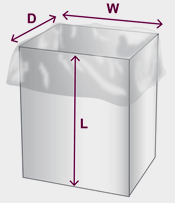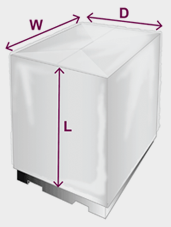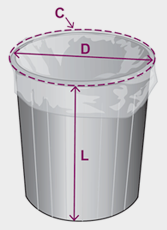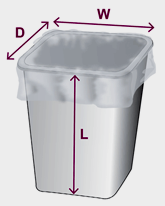# Find the Right Size BagSquare Container - Gusseted Bag

1. Measure the width, depth and height of the box to be lined. The depth should be the shorter side.
2. To determine the Width (W) of the bag, add 1" to the width of the box.
3. To determine the Depth (D) of the bag, add 1" to the depth of the box.
4. The Length (L) of the bag is determined by adding the depth to the height of the box plus 6" to allow coverage of the box contents.

Example

If the box size is 18"(wide) x 12"(deep) x 14"(high), the bag dimensions are as follows: Width (W) is 19" Depth (D) is 13" Length (L) is 32"Pallet Cover - Gusseted Bag

1. Measure the width, depth and height of the pallet to be covered. The depth should be the shorter side.
2. To determine the Width (W) of the cover, add 1"-2" to the width of the pallet.
3. To determine the Depth (D) of the cover, add 1"-2" to the depth of the pallet.
4. The Length (L) of the cover is determined by adding 1/2 the depth to the height.

Example

If the pallet size is 40"(wide) x 36"(deep) x 50"(high), the cover dimensions are as follows: Width (W) is 42" Depth (D) is 38" Length (L) is 68"Rounded Container - Layflat Bag

1. Measure the diameter and height of the container to be lined.
2. To determine the Circumference (C) of the container, multiply the diameter by 3.14.
3. To determine the Width (W) of the bag, divide the Circumference (C) by 2. Add 1" for a looser fit.
4. To determine the Length (L) of the bag, add the diameter to the height. Add 5" for overhang.

Example

If the container size is 12"(diameter) x 30"(height), the bag dimensions are as follows: Width (W) is 20" Length (L) is 47"Square Container - Layflat Bag

1. Measure the width, depth and height of the container to be lined. The depth should be the shorter side.
2. . To determine the Width (W) of the bag, add the width and depth. Add 2" for a looser fit.
3. . The Length (L) of the bag is determined by adding the depth to the height of the box plus 6" to allow coverage of the box contents.

Example

If the container size is 14"(wide) x 10"(deep) x 32"(high), the bag dimensions are as follows: Width (W) is 26" Length (L) is 48"